﻿ Orthogonal Layout
Nevron .NET Vision
Diagram for .NET / User's Guide / Layouts / Graph Parts Layouts / Graph Layouts / Orthogonal Layout

In This Topic
Orthogonal Layout
In This Topic

The orthogonal graph layout is represented by the NOrthogonalGraphLayout class. An orthogonal drawing of a graph is an embedding in the plane such that all edges are drawn as sequences of horizontal and vertical segments. In particular for non­planar and non­biconnected planar graphs, this is a big improvement. The algorithm is complex and very hard to implement, but it is fast and handles both planar and non­planar graphs at the same time.

The algorithm takes as input a simple graph and produces an orthogonal grid drawing. Note that if the input graph is planar, the resulting drawing is also planar (i.e. without edge crossings). In the case of non-planar graphs the edge crossings are inevitable but the algorithm tries to keep their number as low as possible. For both planar and non-planar graphs the layout offers a great improvement in the readability of the graph by maintaining the following aesthetic criteria (given by their priority in descending order):

- minimal number of edge crossings (0 for planar graphs)
- minimal number of bends
- minimal area of the final drawing

Following is a brief description of the algorithm.

Algorithm
In order to produce edge crossing free drawings of planar graphs, we first check if the graph is planar and if it is, then we find a planar embedding of the graph. When planarity testing is completed and the planar topology of the graph is determined, we obtain the faces of the planar graph. Then we compute the orthogonal shape which is an embedding in the plane such that all edges are drawn as sequences of horizontal and vertical line segments. Finally we perform a compaction step, which deals with 2 important tasks: compaction of the total drawing area and bend minimization.
Tuning Considerations

The result of the layout and the aesthetic criteria for the final drawing can be controlled through the following properties:

• UseCompaction – if set to true, a compaction algorithm will be applied to the embedded graph. This will decrease the total area of the drawing with 20 to 50 % (in the average case) at the cost of some additional time needed for the calculations. The following images illustrate the effect of this property in the case of a tree with 13 vertices and 12 edges:

 Orthogonal Graph Layout - No Compaction, Total Area: 7 x 14 = 98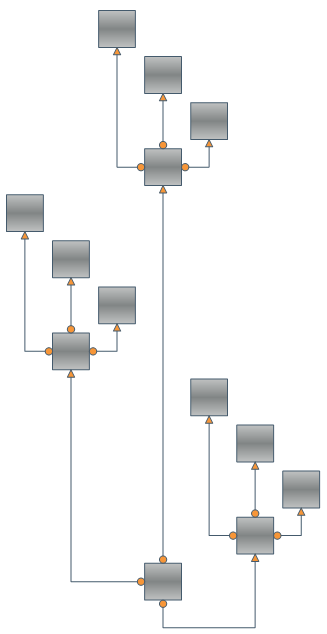Orthogonal Graph Layout – With Compaction, Total Area: 6 x 7 = 42, Compaction Effect: 57%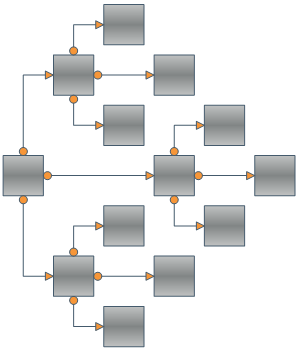• GridCellSizeMode – this property is an enumeration with 2 possible values: GridCellSizeMode.GridBased and GridCellSizeMode.CellBased. If set to the first the maximal size of a node in the graph is determined and all cells are scaled to that size. More area efficient is the second value - it causes the dimensions of each column and row dimensions to be determined according to the size of the cells they contain. See the pictures below for an example of the aforementioned cell sizing scenarios.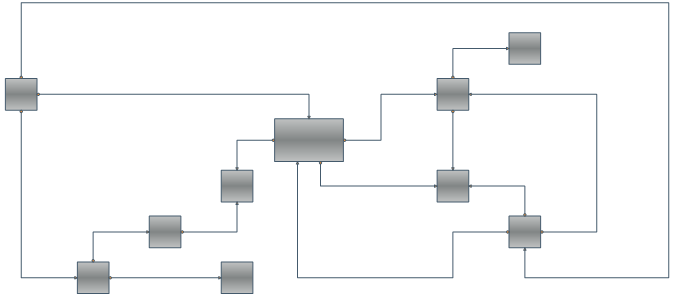Grid based cell size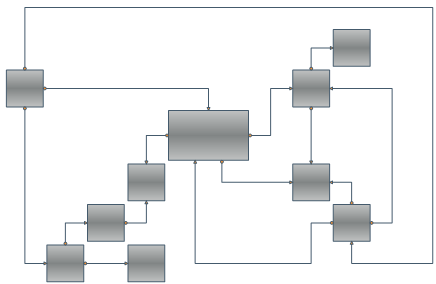Cell based cell size

• CellSpacing – determines the distance between 2 grid cells. For example if a grid cell is calculated to have a size of 100 x 100 and the CellSpacing property is set to 10, then the cell size will be 120 x 120. Note that the node is always placed in the middle of the cell.

Self-loops and duplicate edges

The Orthogonal Graph Layout also handles self-loops and duplicate edges in graphs. It integrates them into the orthogonal grid drawing trying not to increase the total area if possible.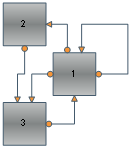Performance Considerations

When layouting very large graphs (i.e. graphs with hundreds or thousands of vertices and edges) the orthogonal graph layout may need more time to execute the complex computations that take part in the layouting process. If you want the layout to complete faster, you can turn off the compaction, but you should be aware of the fact that this will produce a drawing that occupies larger area. You can also set the Multithreaded property to true, because the orthogonal graph layout can take advantage of multicore CPUs.

Related Examples

Windows Forms: Layouts - Orthogonal Layout

Web Forms: Layouts - Orthogonal Layout